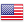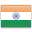Grade "A+" Accredited by NAAC with a CGPA of 3.46
Grade "A+" Accredited by NAAC with a CGPA of 3.46

# Discrete Structure

Course ID
BHCS 04
Level
Program
B.Sc. CS (Hons.)
Semester
Second
Credits
6.0
Paper Type
Core Course
Method
Lecture & Practical

## Unique Paper Code: 32341202

The course aims to introduce the students to Boolean algebra, sets, relations, functions, principles of counting, and growth functions so that these concepts may be used effectively in other courses.

## Learning Outcomes:

At the end of the course, students should be able to:

• Define mathematical structures (relations, functions, sequences, series, and graphs) and use them to model real life situations.
• Understand (trace) and construct simple mathematical proofs using logical arguments.
• Solve class room puzzles based on counting principles.
• Compare functions and relations with respect to their growth for large values of the input.

## Course Contents

Unit 1
Unit 2
Unit 3
Unit 4
Unit 5

Unit 1

Introduction: Sets – finite and infinite sets, uncountable infinite sets; functions, relations, properties of binary relations, closure, partial ordering relations; counting – Pigeonhole Principle, permutation and combination; mathematical induction, Principle of Inclusion and Exclusion.

Unit 2

Growth of Functions: asymptotic notations, summation formulas and properties, bounding summations, approximation by integrals.

Unit 3

Recurrence: recurrence relations, generating functions, linear recurrence relations with constant coefficients and their solution, recursion trees, Master Theorem.

Unit 4

Graph Theory: basic terminology, models and types, multi-graphs and weighted graphs, graph representation, graph isomorphism, connectivity, Euler and Hamiltonian Paths and Circuits, planar graphs, graph coloring, Trees, basic terminology and properties of Trees, introduction to spanning trees.

Unit 5

Propositional Logic: logical connectives, well-formed formulas, tautologies, equivalences, Inference Theory.

### Practicals

#### Lab List 1

1. Write a Program to create a SET A and determine the cardinality of SET for an input array of elements (repetition allowed) and perform the following operations on the SET:
2. a) ismember (a, A): check whether an element belongs to set or not and return value as true/false.
3. b) powerset(A): list all the elements of power set of A.
1. Practical 2
2. Create a class SET and take two sets as input from user to perform following SET Operations:a) Subset: Check whether one set is a subset of other or not.
3. b) Union and Intersection of two Sets.
4. c) Complement: Assume Universal Set as per the input elements from the user.
5. d) Set Difference and Symmetric Difference between two SETS
6. Convert all lowercase characters to uppercase
7. Reverse the string
4. Write a program to merge two ordered arrays to get a single ordered array.
5. Write a program to search a given element in a set of N numbers using Binary search

1. with recursion
2. without recursion
6. Write a program to calculate GCD of two numbers (i) with recursion (ii) without recursion.
7. Create Matrix class. Write a menu-driven program to perform following Matrix operations:

1. Sum
2. Product
3. Transpose
8. Define a class Person having name as a data member. Inherit two classes Student and Employee from Person. Student has additional attributes as course, marks and year and Employee has department and salary. Write display() method in all the three classes to display the corresponding attributes. Provide the necessary methods to show runtime polymorphism.
9. Create a class Triangle. Include overloaded functions for calculating area. Overload assignment operator and equality operator.

#### Lab List 2

1. Write a program to read two numbers p and q. If q is 0 then throw an exception else display the result of p/q.
2. Rewrite Matrix class of Q8 with exception handling. Exceptions should be thrown by the functions if matrices passed to them are incompatible and handled by main() function.
3. Create a class Student containing fields for Roll No., Name, Class, Year and Total Marks. Write a program to store 5 objects of Student class in a file. Retrieve these records from file and display them.
4. Copy the contents of one text file to another file, after removing all whitespaces.

#### Text Books

Mohapatra, & Liu, C. L. (2012). Elements of Discrete mathematics. 4th edition. McGraw Hill Education.
Rosen, K. H. (2011). Discrete Mathematics and Its Applications. 7th edition. Tata McGraw Hill Education.

Albertson, M. O., & Hutchinson, J.P., (1988). Discrete Mathematics with Algorithms. John Wiley and Sons.
Cormen, T. H., Leiserson, C. E., & Rivest, R. L. (2009). Introduction to algorithms. 3rd edition. MIT Press.
Hein, J. L. (2015). Discrete Structures, Logic, and Computability. 4th edition. Jones and Bartlett Learning
Hunter, D. J. (2011). Essentials of Discrete Mathematics. 2nd edition. Jones and Bartlett Learning

#### Teaching Learning Process

Use of ICT tools in conjunction with traditional class room teaching methods
Interactive sessions
Class discussions

#### Assessment Methods

Written tests, assignments, quizzes, presentations as announced by the instructor in the class

#### Keywords

Recurrence, trees and graphs, combinatorics, inductive and deductive reasoning, asymptotic complexity.

Disclaimer: Details on this page are subject to change as per University of Delhi guidelines. For latest update in this regard please refer to the University of Delhi website here.Englishहिन्दी